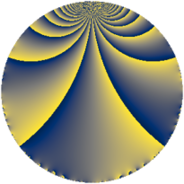# Properties

 Label 7800.2.a.bsLevel $7800$ Weight $2$ Character orbit 7800.a Self dual yes Analytic conductor $62.283$ Analytic rank $0$ Dimension $3$ CM no Inner twists $1$

# Related objects

## Newspace parameters

 Level: $$N$$ $$=$$ $$7800 = 2^{3} \cdot 3 \cdot 5^{2} \cdot 13$$ Weight: $$k$$ $$=$$ $$2$$ Character orbit: $$[\chi]$$ $$=$$ 7800.a (trivial)

## Newform invariants

 Self dual: yes Analytic conductor: $$62.2833135766$$ Analytic rank: $$0$$ Dimension: $$3$$ Coefficient field: 3.3.3732.1 Defining polynomial: $$x^{3} - x^{2} - 13 x + 19$$ Coefficient ring: $$\Z[a_1, \ldots, a_{11}]$$ Coefficient ring index: $$1$$ Twist minimal: yes Fricke sign: $$-1$$ Sato-Tate group: $\mathrm{SU}(2)$

## $q$-expansion

Coefficients of the $$q$$-expansion are expressed in terms of a basis $$1,\beta_1,\beta_2$$ for the coefficient ring described below. We also show the integral $$q$$-expansion of the trace form.

 $$f(q)$$ $$=$$ $$q + q^{3} + \beta_{2} q^{7} + q^{9} +O(q^{10})$$ $$q + q^{3} + \beta_{2} q^{7} + q^{9} + ( 2 - \beta_{1} ) q^{11} - q^{13} + 3 q^{17} + ( -\beta_{1} - \beta_{2} ) q^{19} + \beta_{2} q^{21} + ( -2 + \beta_{1} + \beta_{2} ) q^{23} + q^{27} + ( -1 - 2 \beta_{1} ) q^{29} + ( 2 - \beta_{1} ) q^{31} + ( 2 - \beta_{1} ) q^{33} + ( -2 - \beta_{1} + \beta_{2} ) q^{37} - q^{39} + ( -\beta_{1} + \beta_{2} ) q^{41} + ( -\beta_{1} + \beta_{2} ) q^{43} + ( 4 + 2 \beta_{1} - \beta_{2} ) q^{47} + ( 8 + 3 \beta_{1} - \beta_{2} ) q^{49} + 3 q^{51} + ( -1 + \beta_{1} + \beta_{2} ) q^{53} + ( -\beta_{1} - \beta_{2} ) q^{57} + ( 4 + 2 \beta_{1} - \beta_{2} ) q^{59} + ( 3 + 2 \beta_{1} ) q^{61} + \beta_{2} q^{63} + ( 8 + \beta_{1} ) q^{67} + ( -2 + \beta_{1} + \beta_{2} ) q^{69} + ( 3 \beta_{1} - \beta_{2} ) q^{71} + ( 3 \beta_{1} - \beta_{2} ) q^{73} + ( 1 - 2 \beta_{1} ) q^{77} + ( 4 + \beta_{1} - \beta_{2} ) q^{79} + q^{81} + ( -6 + 2 \beta_{1} + \beta_{2} ) q^{83} + ( -1 - 2 \beta_{1} ) q^{87} + ( 6 - 2 \beta_{1} ) q^{89} -\beta_{2} q^{91} + ( 2 - \beta_{1} ) q^{93} + ( 4 + 2 \beta_{2} ) q^{97} + ( 2 - \beta_{1} ) q^{99} +O(q^{100})$$ $$\operatorname{Tr}(f)(q)$$ $$=$$ $$3q + 3q^{3} + q^{7} + 3q^{9} + O(q^{10})$$ $$3q + 3q^{3} + q^{7} + 3q^{9} + 5q^{11} - 3q^{13} + 9q^{17} - 2q^{19} + q^{21} - 4q^{23} + 3q^{27} - 5q^{29} + 5q^{31} + 5q^{33} - 6q^{37} - 3q^{39} + 13q^{47} + 26q^{49} + 9q^{51} - q^{53} - 2q^{57} + 13q^{59} + 11q^{61} + q^{63} + 25q^{67} - 4q^{69} + 2q^{71} + 2q^{73} + q^{77} + 12q^{79} + 3q^{81} - 15q^{83} - 5q^{87} + 16q^{89} - q^{91} + 5q^{93} + 14q^{97} + 5q^{99} + O(q^{100})$$

Basis of coefficient ring in terms of a root $$\nu$$ of $$x^{3} - x^{2} - 13 x + 19$$:

 $$\beta_{0}$$ $$=$$ $$1$$ $$\beta_{1}$$ $$=$$ $$\nu$$ $$\beta_{2}$$ $$=$$ $$\nu^{2} + \nu - 9$$
 $$1$$ $$=$$ $$\beta_0$$ $$\nu$$ $$=$$ $$\beta_{1}$$ $$\nu^{2}$$ $$=$$ $$\beta_{2} - \beta_{1} + 9$$

## Embeddings

For each embedding $$\iota_m$$ of the coefficient field, the values $$\iota_m(a_n)$$ are shown below.

For more information on an embedded modular form you can click on its label.

Label $$\iota_m(\nu)$$ $$a_{2}$$ $$a_{3}$$ $$a_{4}$$ $$a_{5}$$ $$a_{6}$$ $$a_{7}$$ $$a_{8}$$ $$a_{9}$$ $$a_{10}$$
1.1
 1.56943 −3.77576 3.20633
0 1.00000 0 0 0 −4.96747 0 1.00000 0
1.2 0 1.00000 0 0 0 1.48059 0 1.00000 0
1.3 0 1.00000 0 0 0 4.48688 0 1.00000 0
 $$n$$: e.g. 2-40 or 990-1000 Significant digits: Format: Complex embeddings Normalized embeddings Satake parameters Satake angles

## Atkin-Lehner signs

$$p$$ Sign
$$2$$ $$1$$
$$3$$ $$-1$$
$$5$$ $$1$$
$$13$$ $$1$$

## Inner twists

This newform does not admit any (nontrivial) inner twists.

## Twists

By twisting character orbit
Char Parity Ord Mult Type Twist Min Dim
1.a even 1 1 trivial 7800.2.a.bs yes 3
5.b even 2 1 7800.2.a.bh 3

By twisted newform orbit
Twist Min Dim Char Parity Ord Mult Type
7800.2.a.bh 3 5.b even 2 1
7800.2.a.bs yes 3 1.a even 1 1 trivial

## Hecke kernels

This newform subspace can be constructed as the intersection of the kernels of the following linear operators acting on $$S_{2}^{\mathrm{new}}(\Gamma_0(7800))$$:

 $$T_{7}^{3} - T_{7}^{2} - 23 T_{7} + 33$$ $$T_{11}^{3} - 5 T_{11}^{2} - 5 T_{11} + 3$$ $$T_{17} - 3$$ $$T_{19}^{3} + 2 T_{19}^{2} - 36 T_{19} + 60$$

## Hecke characteristic polynomials

$p$ $F_p(T)$
$2$ $$T^{3}$$
$3$ $$( -1 + T )^{3}$$
$5$ $$T^{3}$$
$7$ $$33 - 23 T - T^{2} + T^{3}$$
$11$ $$3 - 5 T - 5 T^{2} + T^{3}$$
$13$ $$( 1 + T )^{3}$$
$17$ $$( -3 + T )^{3}$$
$19$ $$60 - 36 T + 2 T^{2} + T^{3}$$
$23$ $$-132 - 32 T + 4 T^{2} + T^{3}$$
$29$ $$-201 - 45 T + 5 T^{2} + T^{3}$$
$31$ $$3 - 5 T - 5 T^{2} + T^{3}$$
$37$ $$-20 - 24 T + 6 T^{2} + T^{3}$$
$41$ $$44 - 36 T + T^{3}$$
$43$ $$44 - 36 T + T^{3}$$
$47$ $$361 - 19 T - 13 T^{2} + T^{3}$$
$53$ $$-97 - 37 T + T^{2} + T^{3}$$
$59$ $$361 - 19 T - 13 T^{2} + T^{3}$$
$61$ $$263 - 13 T - 11 T^{2} + T^{3}$$
$67$ $$-453 + 195 T - 25 T^{2} + T^{3}$$
$71$ $$636 - 140 T - 2 T^{2} + T^{3}$$
$73$ $$636 - 140 T - 2 T^{2} + T^{3}$$
$79$ $$36 + 12 T - 12 T^{2} + T^{3}$$
$83$ $$-463 - 3 T + 15 T^{2} + T^{3}$$
$89$ $$16 + 32 T - 16 T^{2} + T^{3}$$
$97$ $$536 - 28 T - 14 T^{2} + T^{3}$$